EnchantedLearning.com is a user-supported site.
As a bonus, site members have access to a banner-ad-free version of the site, with print-friendly pages.

 You might also like: Visual Division #2 Worksheet Printout Visual Division #4 Worksheet Printout Divide Fruit into Two Groups Worksheet Printout Divide Fruit into Three Groups Worksheet Printout Visual Division #3 Worksheet Printout Today's featured page: Christmas Crafts for Kids

 More Math Activities EnchantedLearning.com Visual Division Worksheets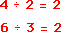More Division

Learn the basics of division using pictures of objects that are divided into groups.If you have 4 fish and want to divide them equally into 2 tanks, you will have 2 tanks in each tank.

The following are printable visual division worksheets:Divide and Draw #1 Divide by 2Divide the items into groups to do division. Draw the divided items and write how many there are. Or go to the answers.Divide and Draw #2 Divide by 3Divide the items into groups to do division. Draw the divided items and write how many there are. Or go to the answers.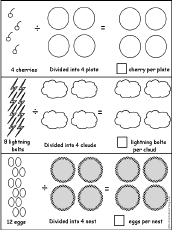Divide and Draw #3Divide by 4Divide the items into groups to do division. Draw the divided items and write how many there are. Or go to the answers.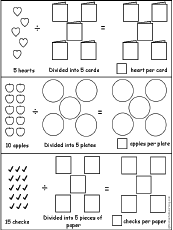Divide and Draw #4Divide by 5Divide the items into groups to do division. Draw the divided items and write how many there are. Or go to the answers.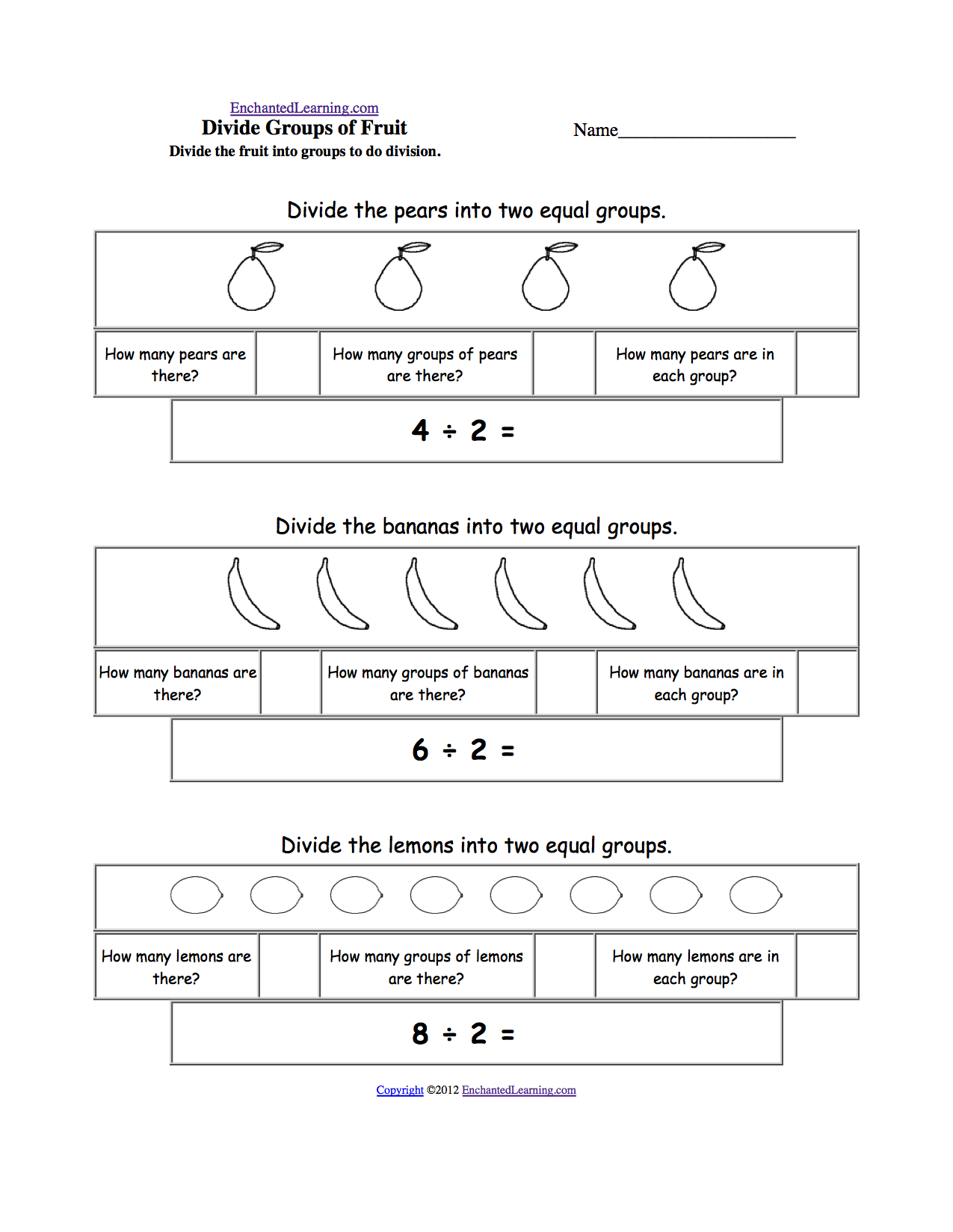Divide Fruit into Two GroupsDividing by two. Divide 4 pears into 2 equal groups (4÷2=2), divide 6 bananas into 2 groups (6÷2=3), then divide 8 lemons into 2 groups (8÷2=4). Or go to the answers.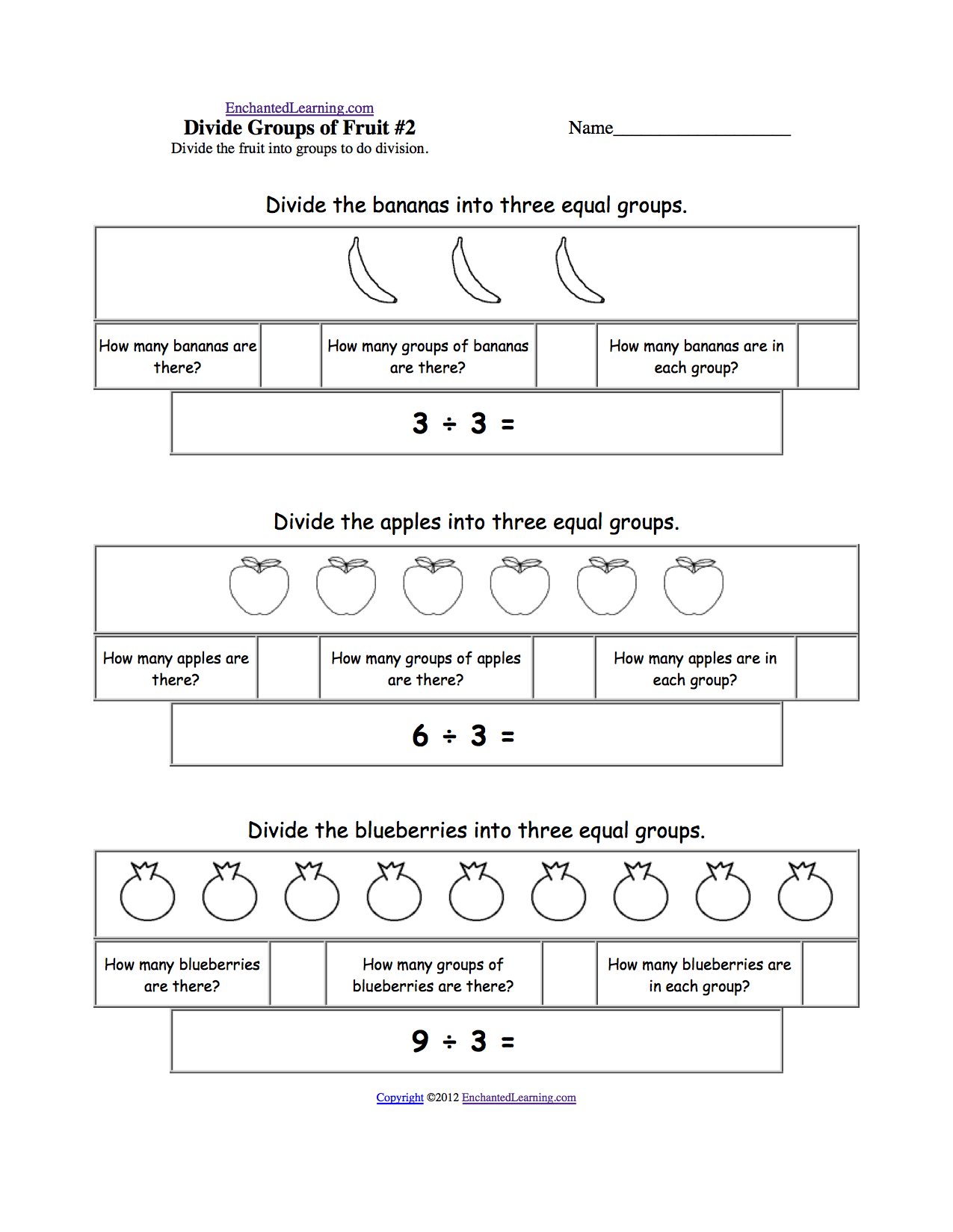Divide Fruit into Three Groups Dividing by three. Divide 3 bananas into 3 equal groups (6÷3=2), divide 6 apples into 3 groups (6÷3=2), then divide 9 blueberries into 3 groups (9÷3=3). Or go to the answers.Divide Fruit into Four GroupsDividing by four. Divide 4 pears into 4 equal groups (4÷4=1), divide 8 oranges into 4 groups (8÷4=2), then divide 12 cherries into 4 groups (12÷4=3). Or go to the answers.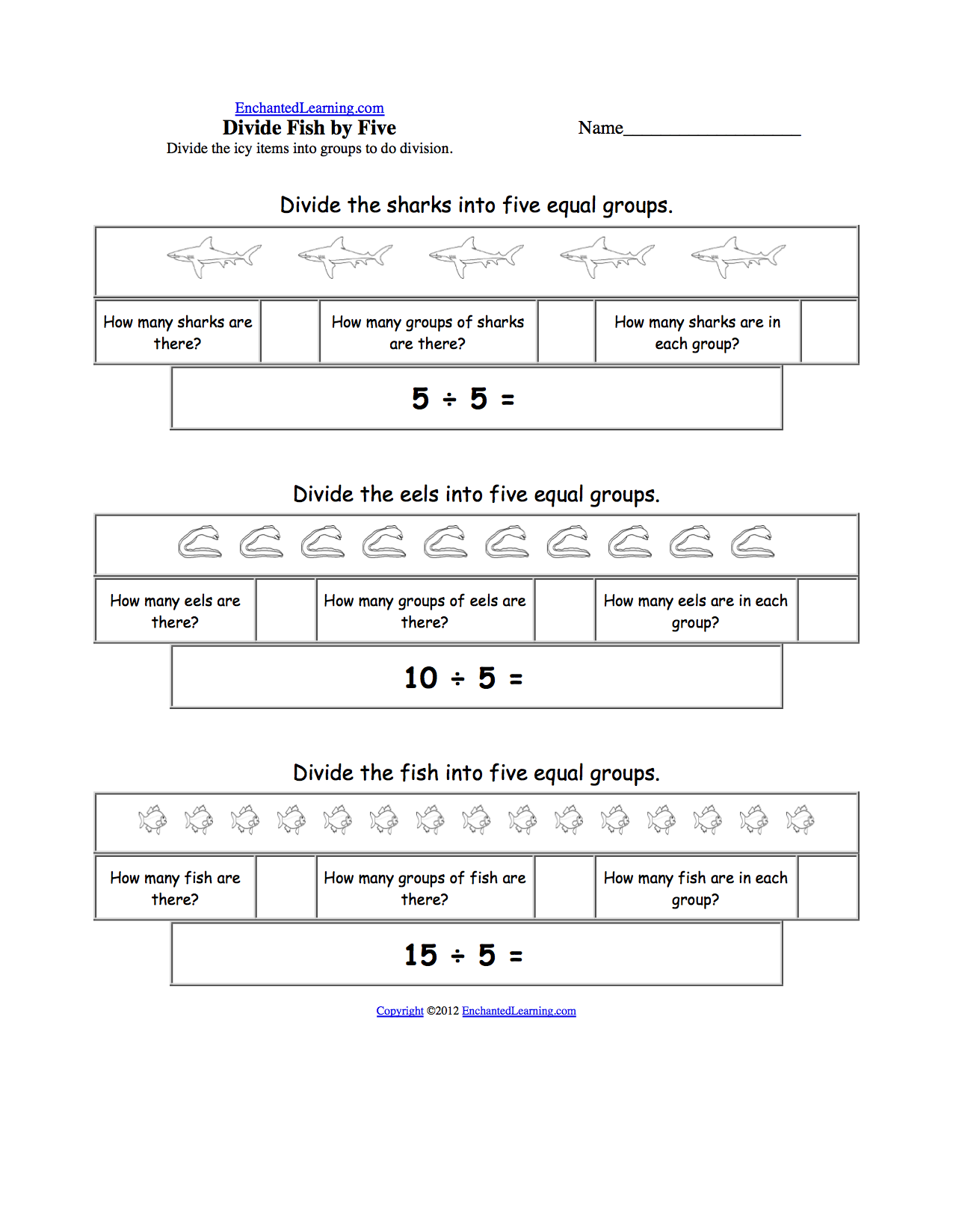Divide Fish into Five GroupsDividing by five. Divide 5 sharks into 5 equal groups (5÷5=1), divide 10 eels into 5 groups (10÷5=2), then divide 15 fish into 3 groups (15÷3=5). Or go to the answers.Divide Six by 2, 3, and 6Divide 6 balloons into 2 equal groups (6÷2=3), divide 6 gifts into 3 groups (6÷3=2), then divide 6 ice cream cones into 6 groups (6÷6=2). Or go to the answers.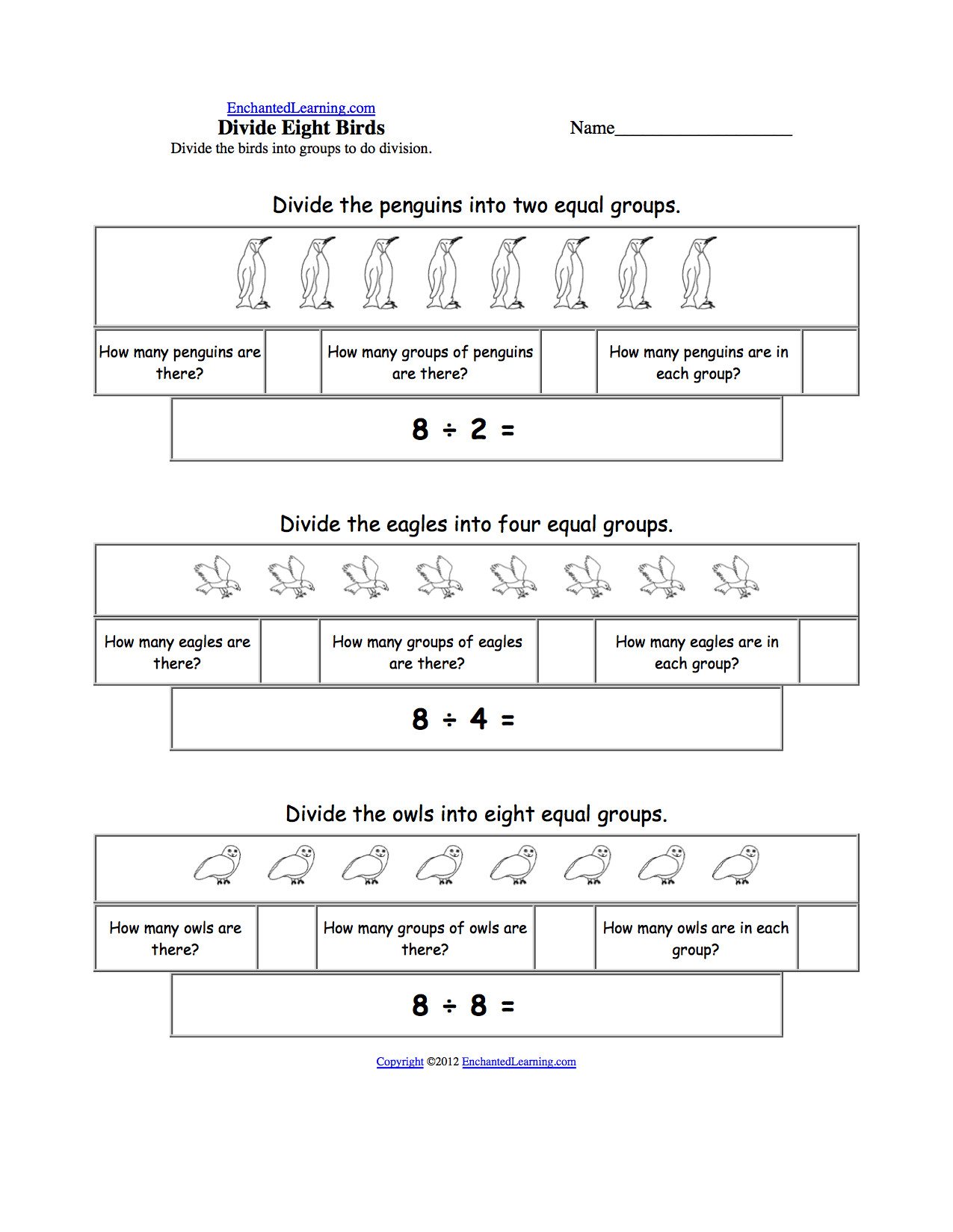Divide Eight Birds by 2, 4, and 8Divide 8 penguins into 2 equal groups (8÷2=4), divide 8 eagles into 4 groups (8÷4=2), then divide 8 owls into 8 groups (8÷8=1). Or go to the answers.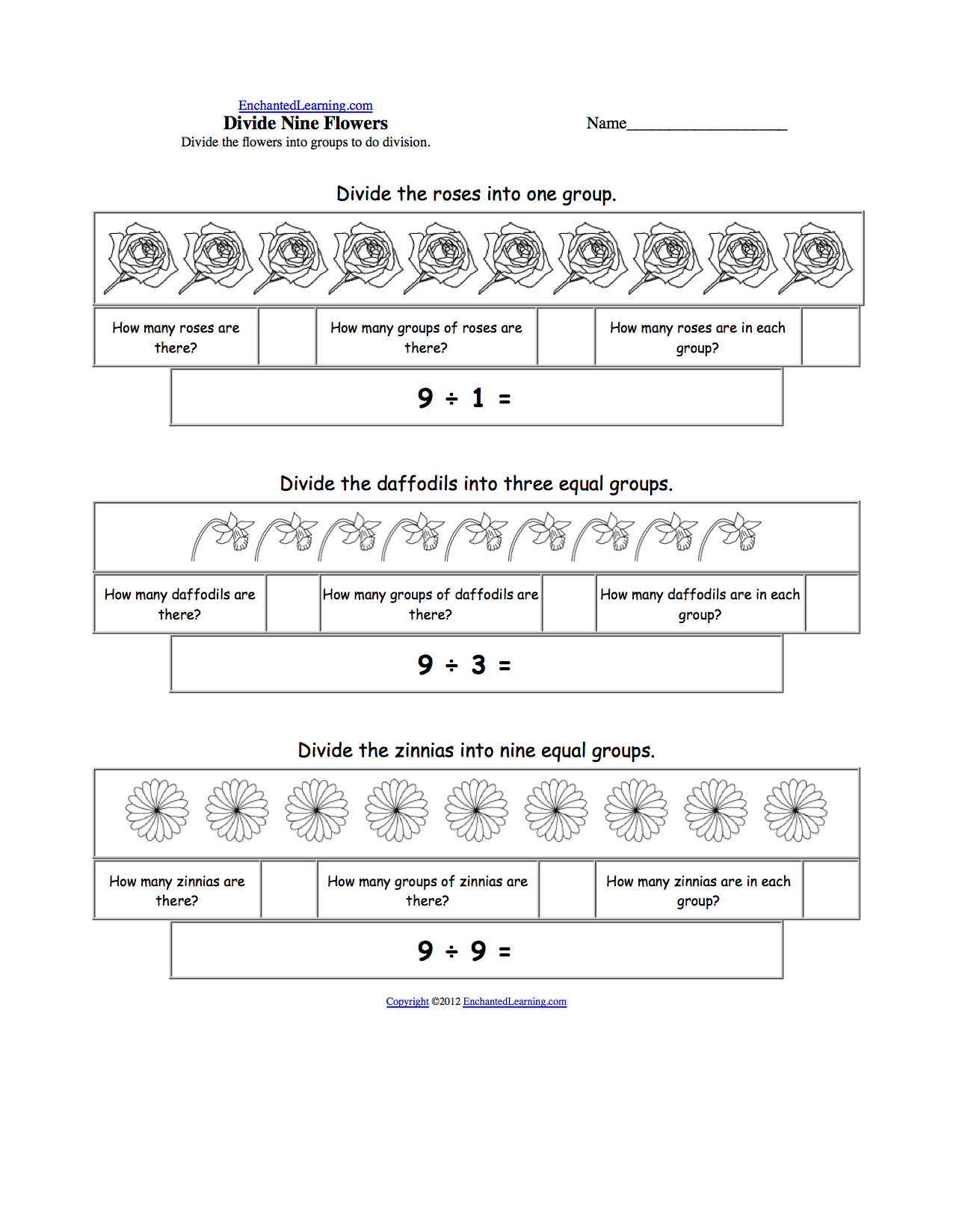Divide Nine Flowers by 1, 3, and 9Divide 9 roses into 1 group (9÷1=9), divide 9 daffodils into 3 groups (9÷3=3), then divide 9 zinnias into 9 groups (9÷9=1). Or go to the answers.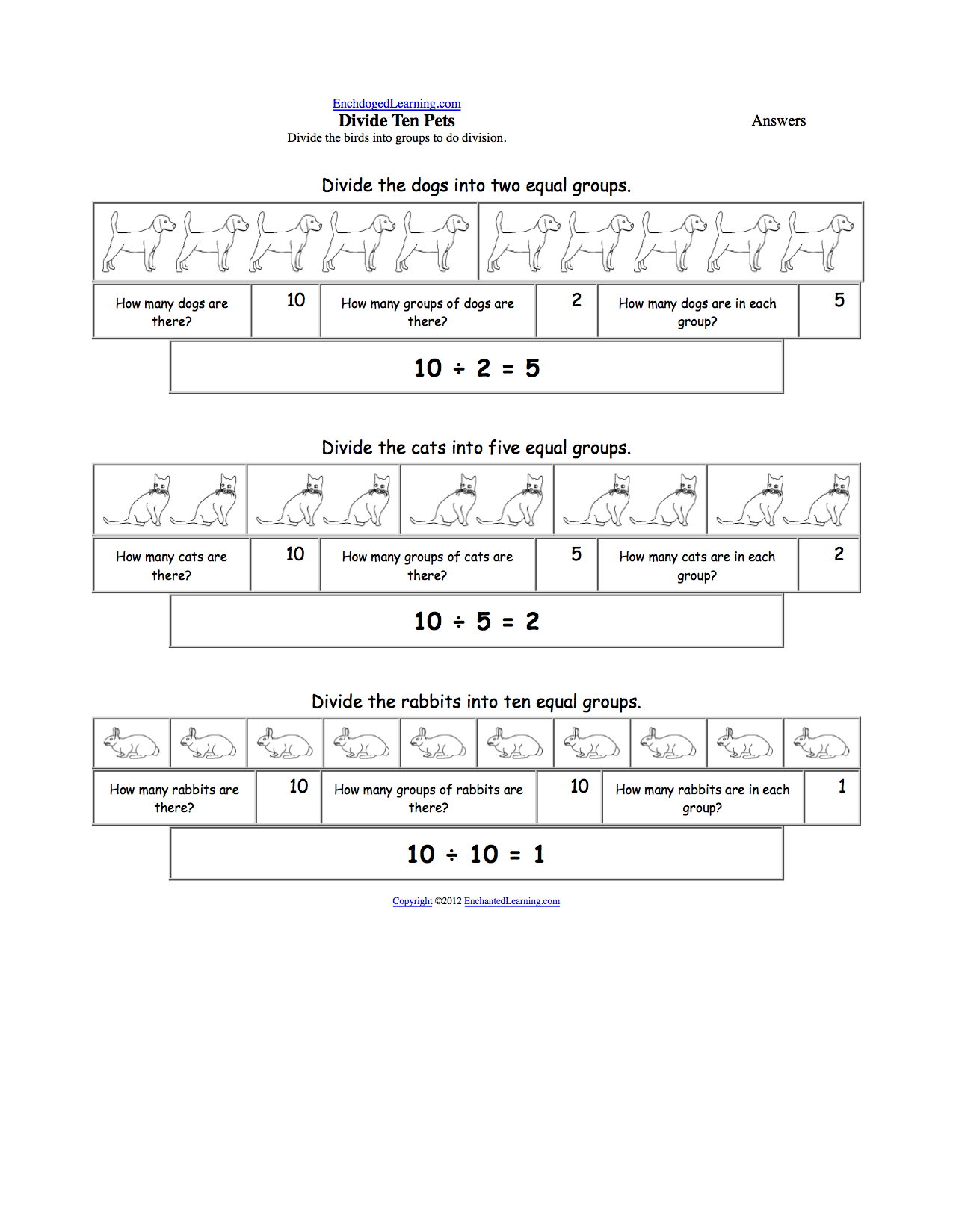Divide Ten Pets by 2, 5, and 10Divide 10 dogs into 2 equal groups (10÷2=5), divide 10 cats into 5 groups (10÷5=2), then divide 10 rabbits into 10 groups (10÷10=1). Or go to the answers.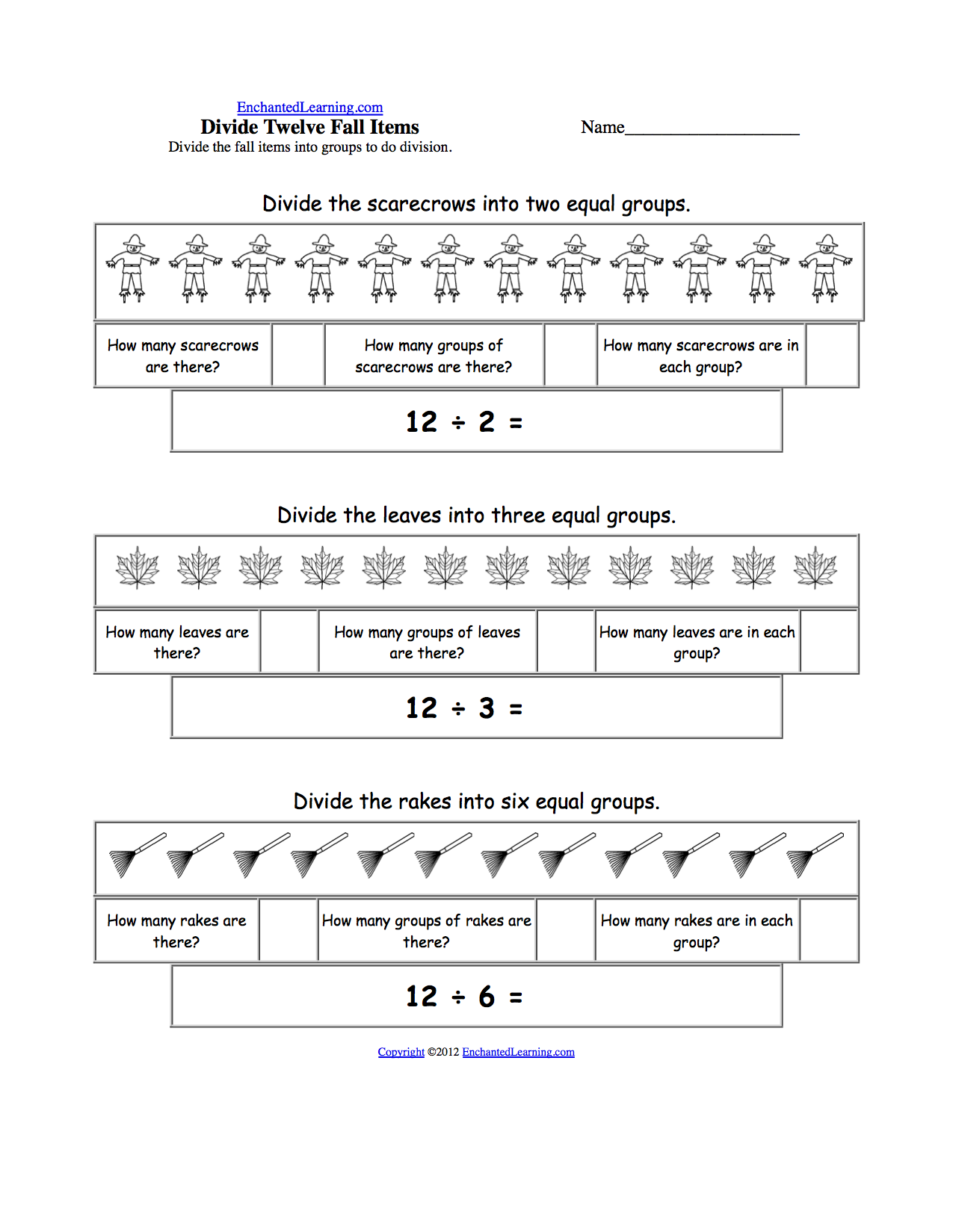Divide Twelve Fall Items by 2, 3, and 6Divide 12 scarecrows into 2 equal groups (12÷2=6), divide 12 leaves into 3 groups (12÷3=4), then divide 12 rakes into 6 groups (12÷6=2). Or go to the answers.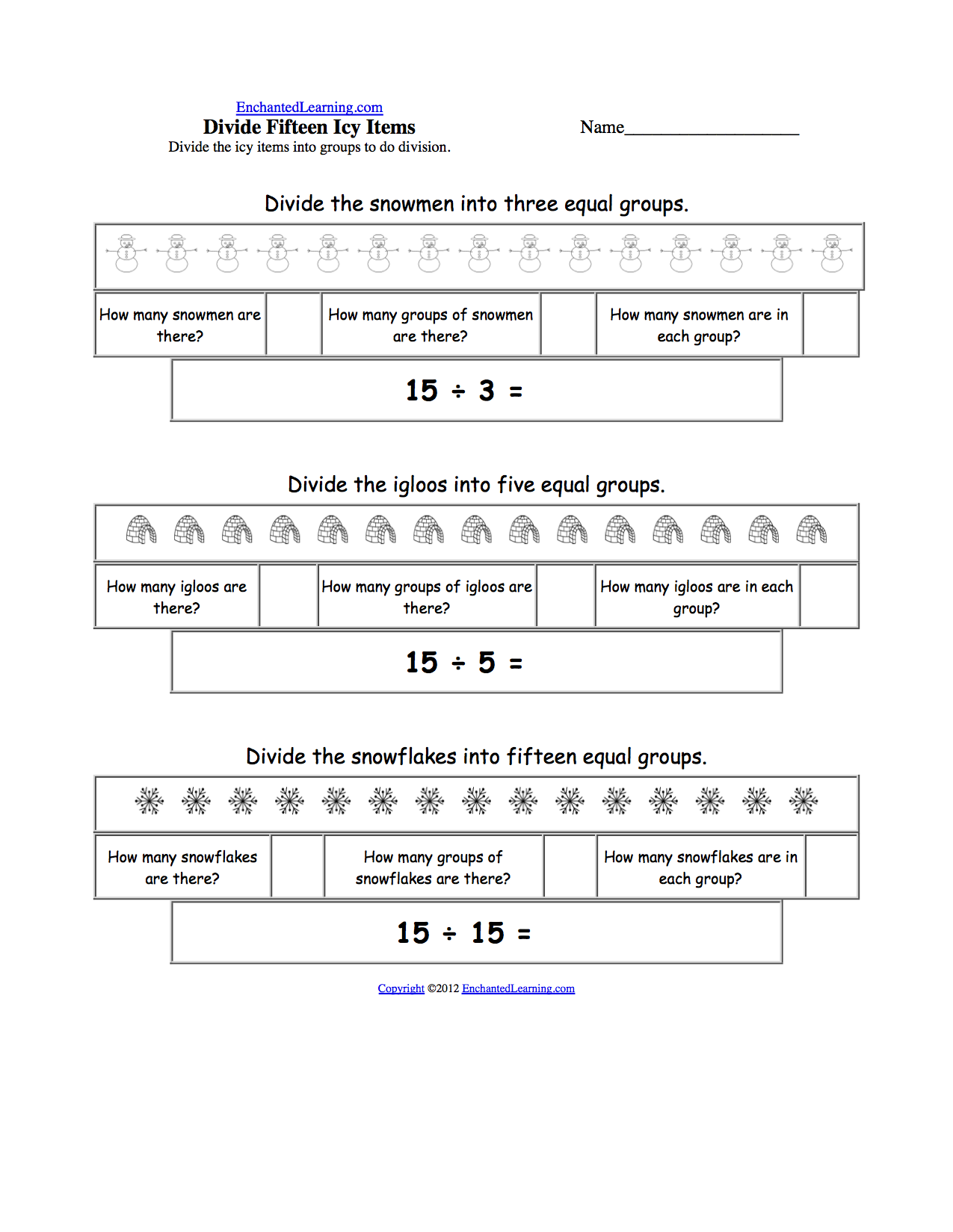Divide Fifteen Icy Items by 3, 5, and 15Divide 15 snowmen into 3 equal groups (15÷3=5), divide 15 igloos into 5 groups (15÷5=3), then divide 15 snowflakes into 15 groups (15÷15=1). Or go to the answers.Divide Sixteen Farm Animals by 2, 4, and 8Divide 16 goats into 2 equal groups (16÷2=8), divide 16 cows into 4 groups (16÷4=4), then divide 16 pigs into 16 groups (16÷8=2). Or go to the answers.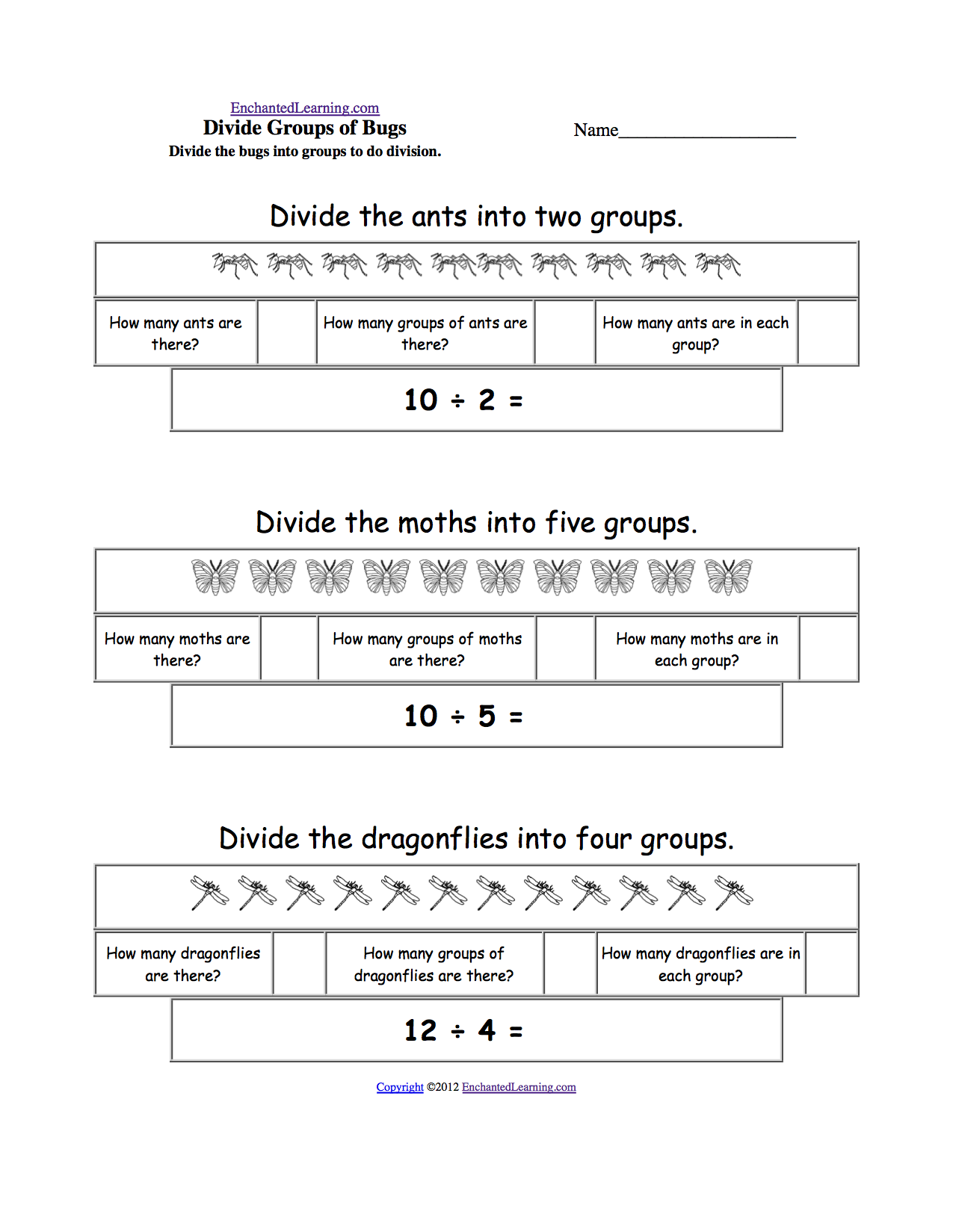Divide Bugs into GroupsDivide 10 ants into 2 equal groups (10÷2=5), divide 10 moths into 5 groups (10÷5=2), then divide 12 dragonflies into 4 groups (12÷4=3). Or go to the answers.

 +, - EnchantedLearning.comMath x, ÷
 A B C D E F G H I J K L M N O P Q R S T U V W X Y Z
 Number Line Fractions Decimals Measurement Rounding Graphs

Enchanted Learning®
Over 35,000 Web Pages
Sample Pages for Prospective Subscribers, or click below

 Overview of Site What's New Enchanted Learning Home Monthly Activity Calendar Books to Print Site Index K-3 Crafts K-3 Themes Little ExplorersPicture dictionary PreK/K Activities Rebus Rhymes Stories Writing Cloze Activities Essay Topics Newspaper Writing Activities Parts of Speech Fiction The Test of Time iPhone app TapQuiz Maps - free iPhone Geography Game Biology Animal Printouts Biology Label Printouts Biomes Birds Butterflies Dinosaurs Food Chain Human Anatomy Mammals Plants Rainforests Sharks Whales Physical Sciences: K-12 Astronomy The Earth Geology Hurricanes Landforms Oceans Tsunami Volcano Languages Dutch French German Italian Japanese (Romaji) Portuguese Spanish Swedish Geography/History Explorers Flags Geography Inventors US History Other Topics Art and Artists Calendars College Finder Crafts Graphic Organizers Label Me! Printouts Math Music Word Wheels

## Enchanted Learning Search

 Search the Enchanted Learning website for: